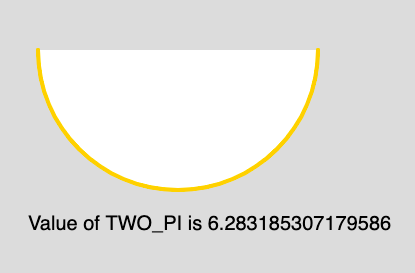Open in App
Not now

# p5.js | Constants | TWO_PI

• Last Updated : 16 Apr, 2019

The TWO_PI is a mathematical constant with the value 6.28318530717958647693. It is twice the ratio of the circumference of a circle to its diameter.

Syntax:

`TWO_PI`

Below program illustrates the usage of TWO_PI in p5.js:

Example:

 `function` `setup() { ` `     `  `    ``// Create Canvas of size 880*300   ` `    ``createCanvas(880, 300); ` `} ` `  `  `function` `draw() { ` `  `  `    ``// Set the Background Color ` `    ``background(220); ` `  `  `    ``// Set the stroke color ` `    ``stroke(255, 204, 0); ` `  `  `    ``// Set the stroke weight ` `    ``strokeWeight(4); ` `  `  `    ``// Draw Arc ` `    ``// Use of constant TWO_PI ` `    ``arc(180, 50, 280, 280, 0, TWO_PI/2); ` `  `  `    ``// Unset the stroke ` `    ``noStroke(); ` `  `  `    ``// Set the text size ` `    ``textSize(20); ` `     `  `    ``// Display output ` `    ``text(``"Value of TWO_PI is "``+ TWO_PI, 30, 230); ` `} `

Output:Reference: https://p5js.org/reference/#/p5/TWO_PI

My Personal Notes arrow_drop_up
Related Articles